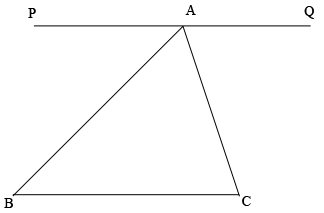SEARCH HOMEMath Central Quandaries & QueriesQuestion from Sophia, a student: Does every triangle add up to 180 degrees? (Such as a unique triangle)Hi Sophia,

In Euclidean Geometry the sum of the measures of the interior angles of a triangle is 180 degrees. This postulate is equivalent to the parallel postulate, for example look at this Wikipedia page. One proof begins with the diagram below where the line segment $PQ,$ through $A$ is parallel to $BC.$Can you complete the proof?
PennyMath Central is supported by the University of Regina and the Imperial Oil Foundation.